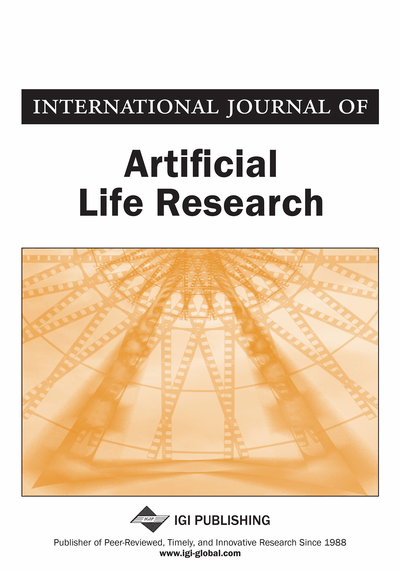# On Quasi Discrete Topological Spaces in Information Systems

Tutut Herawan (Department of Mathematics Education, Universitas Ahmad Dahlan, Yogyakarta, Indonesia)
DOI: 10.4018/jalr.2012040104

## Abstract

This paper presents an alternative way for constructing a topological space in an information system. Rough set theory for reasoning about data in information systems is used to construct the topology. Using the concept of an indiscernibility relation in rough set theory, it is shown that the topology constructed is a quasi-discrete topology. Furthermore, the dependency of attributes is applied for defining finer topology and further characterizing the roughness property of a set. Meanwhile, the notions of base and sub-base of the topology are applied to find attributes reduction and degree of rough membership, respectively.
Article Preview
Top

## Introduction

In the 1980s, Z. Pawlak introduced rough set theory to deal the problem of imprecise knowledge (Pawlak, 1982). Similarly to fuzzy set theory (Zadeh, 1965), it is not an alternative to classical set theory but it is embedded in the set. Fuzzy and rough sets theories are not competitively, but complementary each other (Pawlak & Skowron, 2007). Rough set theory has attracted attention of many researchers and practitioners all over the world, who contributed essentially to its development and applications (Pawlak, 1983, 1997, 2002a, 2002b; Pawlak & Skowron, 2007; Herawan et al., 2009, 2010a, 2010b, 2010c, 2010d; Senan et al., 2011; Yanto et al., 2010a, 2010b, 2011, 2012). The original goal of the rough set theory is induction of approximations of concepts. The idea consists of approximation of a subset by a pair of two precise concepts called the lower approximation and upper approximation (Komorowski et al., 1999). Intuitively, the lower approximation of a set consists of all elements that surely belong to the set, whereas the upper approximation of the set constitutes of all elements that possibly belong to the set. The difference of the upper approximation and the lower approximation is a boundary region. It consists of all elements that cannot be classified uniquely to the set or its complement, by employing available knowledge. Thus any rough set, in contrast to a crisp set, has a non-empty boundary region. Motivation for rough set theory has come from the need to represent a subset of a universe in terms of equivalence classes of a partition of the universe.

The concept of topology shows up naturally in almost every branch of mathematics (Munkers, 1975). This has made topology one of the great unifying ideas of mathematics. Rough set theory may therefore be considered as a method for constructing a topological space using indiscernibility relation on the universe. In a reverse process, we can generalize the notions of rough sets based on the topological space (Herawan & Deris, 2009). Definable sets, i.e. a union of one or more equivalence classes are substituted by open sets in defining the lower approximations, and by closed sets in defining upper approximations (Yao, 1998, 2001). Lashin et al. (2005) presented rough set theory in general topological space and described rough set theory in the topology of binary relation by defining (right) neighborhood and investigate the knowledge representations (granular structures) and processing of binary relations in the style of rough set theory. Zhu (2007) discussed covering-based rough sets from the topological view, defined a new type of covering-based rough sets from a topological concept called neighborhood and established axiomatic systems for the lower and the upper approximations operations. Allam et al. (2008) proposed and discussed some methods for generating topologies are using binary relations. They also investigated some properties of these topologies and obtained a quasi-discrete topology from a symmetric relation instead of an equivalence relation. Zhang et al. (2010) investigated the role of topological De Morgan algebra in the theory of rough sets.

## Complete Article List

Search this Journal:
Reset
Open Access Articles: Forthcoming
Volume 8: 2 Issues (2018)
Volume 7: 2 Issues (2017)
Volume 6: 2 Issues (2016)
Volume 5: 1 Issue (2015)
Volume 4: 1 Issue (2014)
Volume 3: 4 Issues (2012)
Volume 2: 4 Issues (2011)
Volume 1: 4 Issues (2010)
View Complete Journal Contents Listing